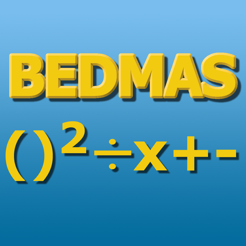Daily Work‎ > ‎

Week of Sept. 17-21

posted Sep 17, 2018, 6:58 AM by Mr. Ingram   [ updated Sep 25, 2018, 6:14 AM ]MONDAY     7A MATH - We learned about powers and exponents today.  Students practiced questions with partners and then worked on page 18 out of our textbooks.     7B MATH - We learned about square roots and how they are the opposite of squaring numbers.  We then reviewed the order of operations - B E DM AS.  Students followed along while some questions were solved on the Smartboard for the class and they then attempted some on their own.  This is a VERY important area and some students are struggling - I will spend several classes on review and practice.     7C MATH - We learned about square roots and how they are the opposite of squaring numbers.  We then reviewed the order of operations - B E DM AS.  Students followed along while some questions were solved on the Smartboard for the class and they then attempted some on their own.  This is a VERY important area and some students are struggling - I will spend several classes on review and practice.     TUESDAY     8C SCIENCE - We focused on learning all the terms and definitions in our Water Systems unit today.  Students used laptops and accessed my Quizlets (link is on the left side of this site) to practice.  At the end of class, students worked in teams with a fun Quizlet Live game.     7B MATH - Students continued to work in partners to solved B E DM AS questions.  A few students did not finish in class and had to finish at home.     7A MATH - We learned about square roots and how they are the opposite of squaring numbers.  We then reviewed the order of operations - B E DM AS.  Students followed along while some questions were solved on the Smartboard for the class and they then attempted some on their own.  This is a VERY important area and some students are struggling - I will spend several classes on review and practice.     WEDNESDAY     8C SCIENCE - Today was our last day for practicing and reviewing terms and definitions.  Students have a quiz tomorrow on definitions.  It is best if they practice online or use the sheets I have sent home.7B MATH - Students worked in partners on three step (only add, subtract, multiply, and divide) equations and then worked on a few four step (including exponents) equations if they finished early.    7C MATH - Students worked in partners on three step (only add, subtract, multiply, and divide) equations and then worked on a few four step (including exponents) equations if they finished early.    7A MATH - Students worked in partners on three step (only add, subtract, multiply, and divide) equations and then worked on a few four step (including exponents) equations if they finished early.    THURSDAY     7C MATH - Students wrote a practice test for BEDMAS, exponents, and prime numbers.  We took up the questions in class together.  I will look over the practice quizzes to see how students are doing and return them early next week.  Students need to bring these practice quizzes home and get them signed.     7B MATH - Students wrote a practice test for BEDMAS, exponents, and prime numbers.  We took up the questions in class together.  I will look over the practice quizzes to see how students are doing and return them early next week.  Students need to bring these practice quizzes home and get them signed.     7A MATH - Students wrote a practice test for BEDMAS, exponents, and prime numbers.  We took up the questions in class together.  I will look over the practice quizzes to see how students are doing and return them early next week.  Students need to bring these practice quizzes home and get them signed.     8C SCIENCE - Students wrote their definition quiz in class today.  If anyone did poorly they can rewrite it on Monday (second NB).     FRIDAY     7B MATH - Students worked on a practice unit test in partners.  It is a worksheet that covers all topics so far.  We will be taking it up together on Monday in class.     7C MATH - Students worked on a practice unit test in partners.  It is a worksheet that covers all topics so far.  We will be taking it up together on Monday in class.     8C SCIENCE - Students learned about watersheds, surface water, and climate.  We watched some videos from my site together and then students worked in partners to answer questions.